# Linear regression analysis in Excel

The tutorial explains the basics of regression analysis and shows a few different ways to do linear regression in Excel.

Imagine this: you are provided with a whole lot of different data and are asked to predict next year's sales numbers for your company. You have discovered dozens, perhaps even hundreds, of factors that can possibly affect the numbers. But how do you know which ones are really important? Run regression analysis in Excel. It will give you an answer to this and many more questions: Which factors matter and which can be ignored? How closely are these factors related to each other? And how certain can you be about the predictions?

## Regression analysis in Excel - the basics

In statistical modeling, regression analysis is used to estimate the relationships between two or more variables:

Dependent variable (aka criterion variable) is the main factor you are trying to understand and predict.

Independent variables (aka explanatory variables, or predictors) are the factors that might influence the dependent variable.

Regression analysis helps you understand how the dependent variable changes when one of the independent variables varies and allows to mathematically determine which of those variables really has an impact.

Technically, a regression analysis model is based on the sum of squares, which is a mathematical way to find the dispersion of data points.  The goal of a model is to get the smallest possible sum of squares and draw a line that comes closest to the data.

In statistics, they differentiate between a simple and multiple linear regression. Simple linear regression models the relationship between a dependent variable and one independent variables using a linear function. If you use two or more explanatory variables to predict the dependent variable, you deal with multiple linear regression. If the dependent variable is modeled as a non-linear function because the data relationships do not follow a straight line, use nonlinear regression instead. The focus of this tutorial will be on a simple linear regression.

As an example, let's take sales numbers for umbrellas for the last 24 months and find out the average monthly rainfall for the same period. Plot this information on a chart, and the regression line will demonstrate the relationship between the independent variable (rainfall) and dependent variable (umbrella sales):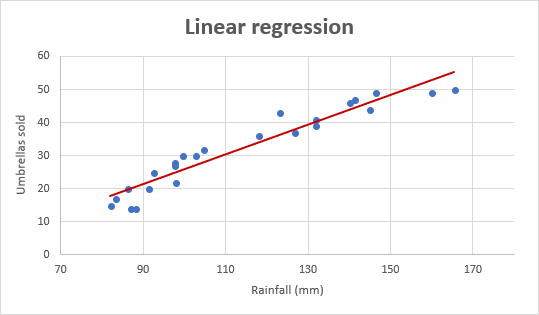### Linear regression equation

Mathematically, a linear regression is defined by this equation:

y = bx + a + ε

Where:

• x is an independent variable.
• y is a dependent variable.
• a is the Y-intercept, which is the expected mean value of y when all x variables are equal to 0. On a regression graph, it's the point where the line crosses the Y axis.
• b is the slope of a regression line, which is the rate of change for y as x changes.
• ε is the random error term, which is the difference between the actual value of a dependent variable and its predicted value.

The linear regression equation always has an error term because, in real life, predictors are never perfectly precise. However, some programs, including Excel, do the error term calculation behind the scenes. So, in Excel, you do linear regression using the least squares method and seek coefficients a and b such that:

y = bx + a

For our example, the linear regression equation takes the following shape:

`Umbrellas sold = b * rainfall + a`

There exist a handful of different ways to find a and b. The three main methods to perform linear regression analysis in Excel are:

• Regression tool included with Analysis ToolPak
• Scatter chart with a trendline
• Linear regression formula

Below you will find the detailed instructions on using each method.

## How to do linear regression in Excel with Analysis ToolPak

This example shows how to run regression in Excel by using a special tool included with the Analysis ToolPak add-in.

### Enable the Analysis ToolPak add-in

Analysis ToolPak is available in all versions of Excel 2019 to 2003 but is not enabled by default. So, you need to turn it on manually. Here's how:

1. In your Excel, click File > Options.
2. In the Excel Options dialog box, select Add-ins on the left sidebar, make sure Excel Add-ins is selected in the Manage box, and click Go.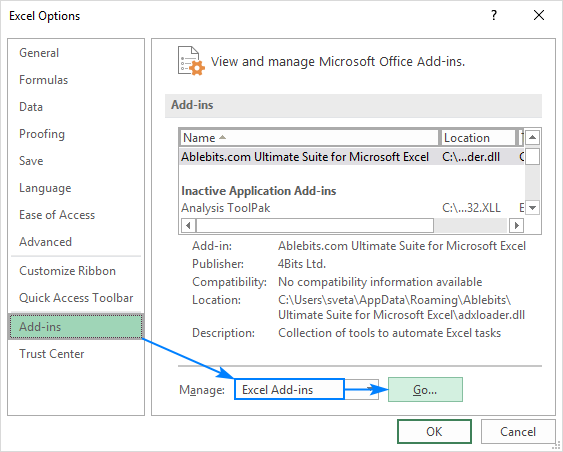3. In the Add-ins dialog box, tick off Analysis Toolpak, and click OK: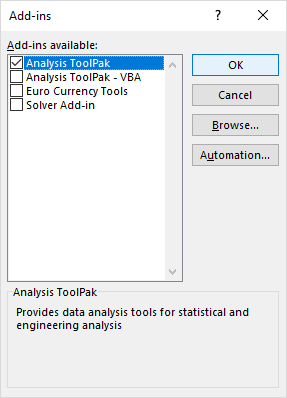This will add the Data Analysis tools to the Data tab of your Excel ribbon.

### Run regression analysis

In this example, we are going to do a simple linear regression in Excel. What we have is a list of average monthly rainfall for the last 24 months in column B, which is our independent variable (predictor), and the number of umbrellas sold in column C, which is the dependent variable. Of course, there are many other factors that can affect sales, but for now we focus only on these two variables: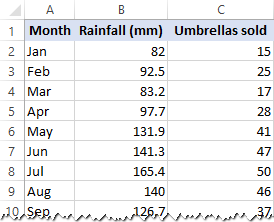With Analysis Toolpak added enabled, carry out these steps to perform regression analysis in Excel:

1. On the Data tab, in the Analysis group, click the Data Analysis button.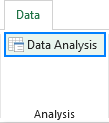2. Select Regression and click OK.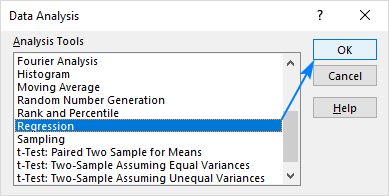3. In the Regression dialog box, configure the following settings:
• Select the Input Y Range, which is your dependent variable. In our case, it's umbrella sales (C1:C25).
• Select the Input X Range, i.e. your independent variable. In this example, it's the average monthly rainfall (B1:B25).

If you are building a multiple regression model, select two or more adjacent columns with different independent variables.

• Check the Labels box if there are headers at the top of your X and Y ranges.
• Choose your preferred Output option, a new worksheet in our case.
• Optionally, select the Residuals checkbox to get the difference between the predicted and actual values.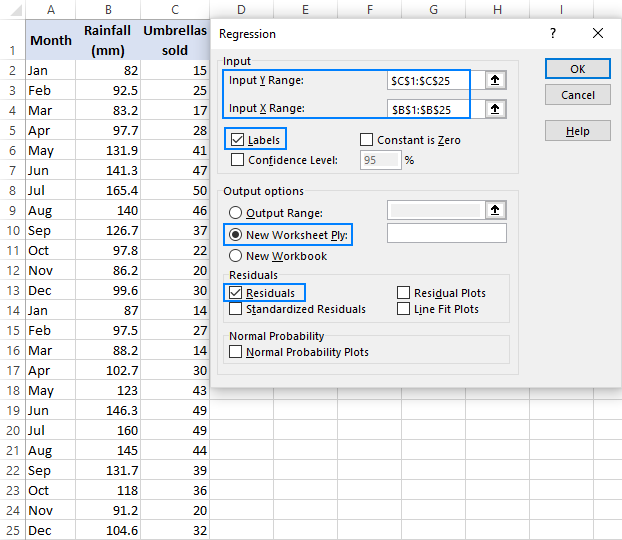4. Click OK and observe the regression analysis output created by Excel.

### Interpret regression analysis output

As you have just seen, running regression in Excel is easy because all calculations are preformed automatically. The interpretation of the results is a bit trickier because you need to know what is behind each number. Below you will find a breakdown of 4 major parts of the regression analysis output.

#### Regression analysis output: Summary Output

This part tells you how well the calculated linear regression equation fits your source data.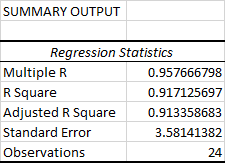Here's what each piece of information means:

Multiple R. It is the Correlation Coefficient that measures the strength of a linear relationship between two variables. The correlation coefficient can be any value between -1 and 1, and its absolute value indicates the relationship strength. The larger the absolute value, the stronger the relationship:

• 1 means a strong positive relationship
• -1 means a strong negative relationship
• 0 means no relationship at all

R Square. It is the Coefficient of Determination, which is used as an indicator of the goodness of fit. It shows how many points fall on the regression line. The R2 value is calculated from the total sum of squares, more precisely, it is the sum of the squared deviations of the original data from the mean.

In our example, R2 is 0.91 (rounded to 2 digits), which is fairy good. It means that 91% of our values fit the regression analysis model. In other words, 91% of the dependent variables (y-values) are explained by the independent variables (x-values). Generally, R Squared of 95% or more is considered a good fit.

Adjusted R Square. It is the R square adjusted for the number of independent variable in the model. You will want to use this value instead of R square for multiple regression analysis.

Standard Error. It is another goodness-of-fit measure that shows the precision of your regression analysis - the smaller the number, the more certain you can be about your regression equation. While R2 represents the percentage of the dependent variables variance that is explained by the model, Standard Error is an absolute measure that shows the average distance that the data points fall from the regression line.

Observations. It is simply the number of observations in your model.

#### Regression analysis output: ANOVA

The second part of the output is Analysis of Variance (ANOVA):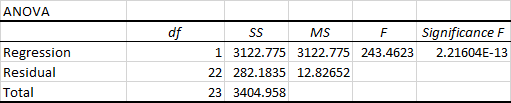Basically, it splits the sum of squares into individual components that give information about the levels of variability within your regression model:

• df is the number of the degrees of freedom associated with the sources of variance.
• SS is the sum of squares. The smaller the Residual SS compared with the Total SS, the better your model fits the data.
• MS is the mean square.
• F is the F statistic, or F-test for the null hypothesis. It is used to test the overall significance of the model.
• Significance F is the P-value of F.

The ANOVA part is rarely used for a simple linear regression analysis in Excel, but you should definitely have a close look at the last component. The Significance F value gives an idea of how reliable (statistically significant) your results are. If Significance F is less than 0.05 (5%), your model is OK. If it is greater than 0.05, you'd probably better choose another independent variable.

#### Regression analysis output: coefficients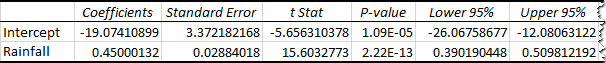The most useful component in this section is Coefficients. It enables you to build a linear regression equation in Excel:

y = bx + a

For our data set, where y is the number of umbrellas sold and x is an average monthly rainfall, our linear regression formula goes as follows:

`Y = Rainfall Coefficient * x + Intercept`

Equipped with a and b values rounded to three decimal places, it turns into:

`Y=0.45*x-19.074`

For example, with the average monthly rainfall equal to 82 mm, the umbrella sales would be approximately 17.8:

`0.45*82-19.074=17.8`

In a similar manner, you can find out how many umbrellas are going to be sold with any other monthly rainfall (x variable) you specify.

#### Regression analysis output: residuals

If you compare the estimated and actual number of sold umbrellas corresponding to the monthly rainfall of 82 mm, you will see that these numbers are slightly different:

• Estimated: 17.8 (calculated above)
• Actual: 15 (row 2 of the source data)

Why's the difference? Because independent variables are never perfect predictors of the dependent variables. And the residuals can help you understand how far away the actual values are from the predicted values: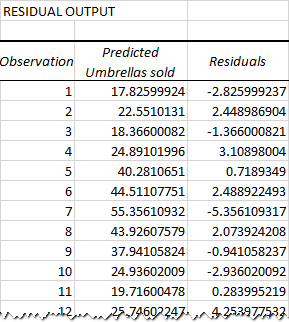For the first data point (rainfall of 82 mm), the residual is approximately -2.8.  So, we add this number to the predicted value, and get the actual value: 17.8 - 2.8 = 15.

## How to make a linear regression graph in Excel

If you need to quickly visualize the relationship between the two variables, draw a linear regression chart. That's very easy! Here's how:

2. On the Inset tab, in the Chats group, click the Scatter chart icon, and select the Scatter thumbnail (the first one):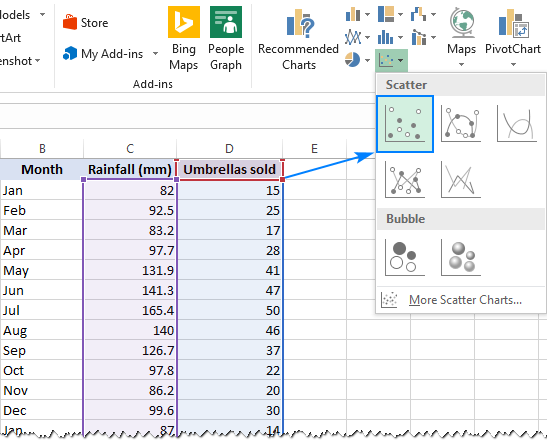This will insert a scatter plot in your worksheet, which will resemble this one: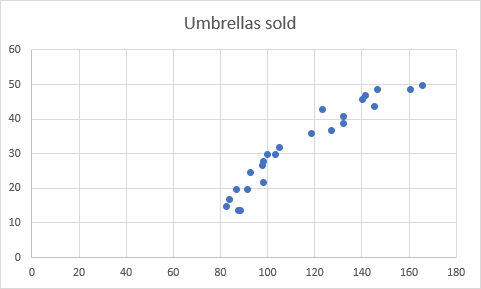3. Now, we need to draw the least squares regression line. To have it done, right click on any point and choose Add Trendline… from the context menu.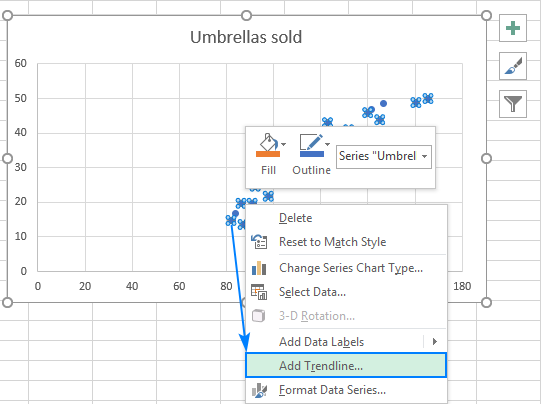4. On the right pane, select the Linear trendline shape and, optionally, check Display Equation on Chart to get your regression formula: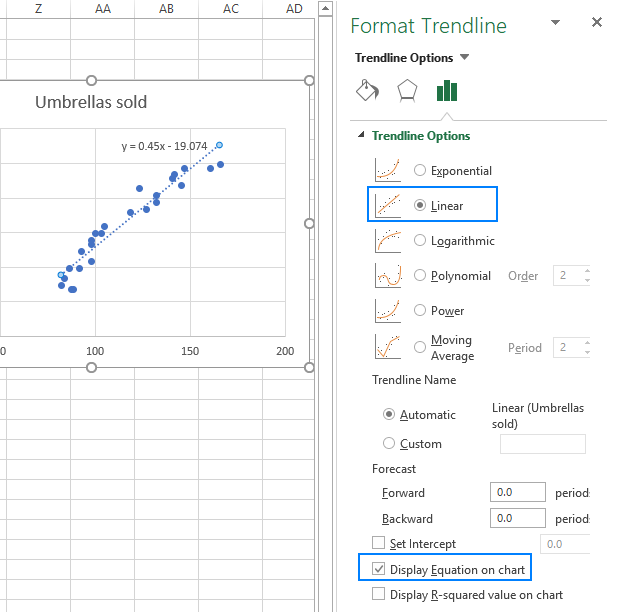As you may notice, the regression equation Excel has created for us is the same as the linear regression formula we built based on the Coefficients output.

5. Switch to the Fill & Line tab and customize the line to your liking. For example, you can choose a different line color and use a solid line instead of a dashed line (select Solid line in the Dash type box):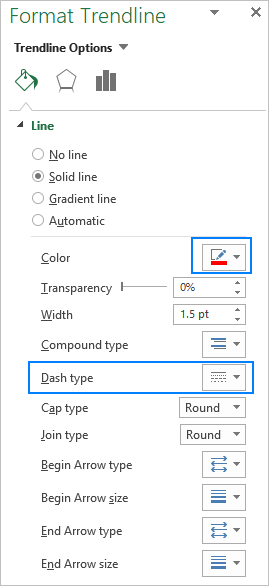At this point, your chart already looks like a decent regression graph: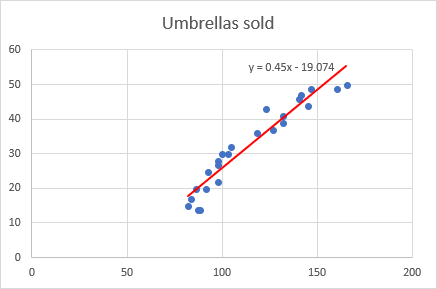Still, you may want to make a few more improvements:

• Drag the equation wherever you see fit.
• Add axes titles (Chart Elements button > Axis Titles).
• If your data points start in the middle of the horizontal and/or vertical axis like in this example, you may want to get rid of the excessive white space. The following tip explains how to do this: Scale the chart axes to reduce white space.

And this is how our improved regression graph looks like: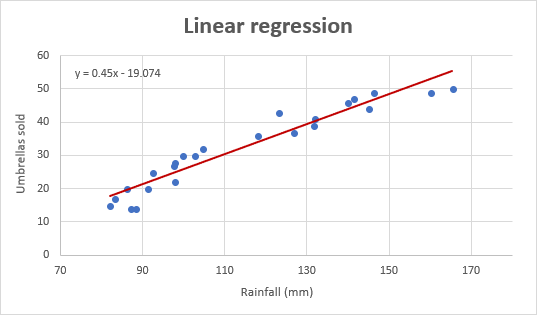Important note! In the regression graph, the independent variable should always be on the X axis and the dependent variable on the Y axis. If your graph is plotted in the reverse order, swap the columns in your worksheet, and then draw the chart anew. If you are not allowed to rearrange the source data, then you can switch the X and Y axes directly in a chart.

## How to do regression in Excel using formulas

Microsoft Excel has a few statistical functions that can help you to do linear regression analysis such as LINEST, SLOPE, INTERCPET, and CORREL.

The LINEST function uses the least squares regression method to calculate a straight line that best explains the relationship between your variables and returns an array describing that line. You can find the detailed explanation of the function's syntax in this tutorial. For now, let's just make a formula for our sample dataset:

`=LINEST(C2:C25, B2:B25)`

Because the LINEST function returns an array of values, you must enter it as an array formula. Select two adjacent cells in the same row, E2:F2 in our case, type the formula, and press Ctrl + Shift + Enter to complete it.

The formula returns the b coefficient (E1) and the a constant (F1) for the already familiar linear regression equation:

`y = bx + a`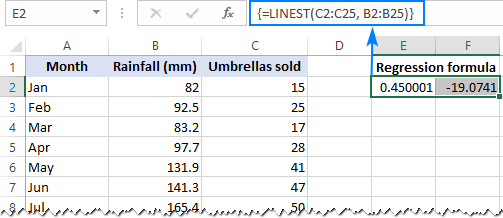If you avoid using array formulas in your worksheets, you can calculate a and b individually with regular formulas:

Get the Y-intercept (a):

`=INTERCEPT(C2:C25, B2:B25)`

Get the slope (b):

`=SLOPE(C2:C25, B2:B25)`

Additionally, you can find the correlation coefficient (Multiple R in the regression analysis summary output) that indicates how strongly the two variables are related to each other:

`=CORREL(B2:B25,C2:C25)`

The following screenshot shows all these Excel regression formulas in action: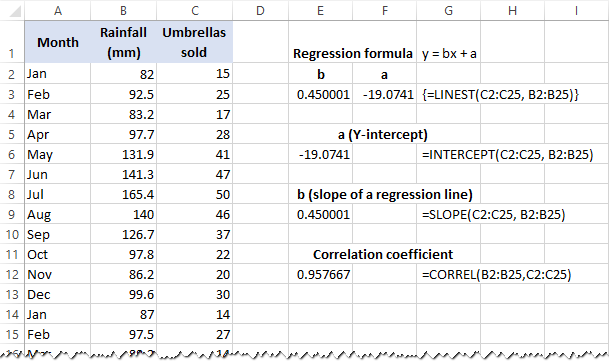Tip. If you'd like to get additional statistics for your regression analysis, use the LINEST function with the stats parameter set to TRUE as shown in this example.

That's how you do linear regression in Excel. That said, please keep in mind that Microsoft Excel is not a statistical program. If you need to perform regression analysis at the professional level, you may want to use targeted software such as XLSTAT, RegressIt, etc.

To have a closer look at our linear regression formulas and other techniques discussed in this tutorial, you are welcome to download our sample Regression Analysis in Excel workbook.

### 66 Responses to "Linear regression analysis in Excel"

1.isaac rono says:

2.Jane says:

3.Julius says:

Thanks! Good easy to follow guide.

4.Shankar Iyer says:

5.Shankar Iyer says:

Thanks Ms.Svetlana for the quick response. Your tutorial was very easy to understand as it went step by step, hand holding a novice..which is very important..! I really appreciate your effort in making complex issues simple. Do you have similar tutorial on Multiple regression, Pricing optimization ,Price bundling etc( in Excel), Decision tree Analysis etc.
Regards
Shankar

6.santhosh says:

The article is very nice keep posting

7.Gleiza says:

hi! the article is very helpful, just a quick question, why is it that i cannot add a trendline? :( pls help

8.wondim says says:

thank you ms. for your help

9.ali says:

very useful guide.thanks

10.Heather Davis says:

So I completed the regression analysis for my assignment. So how do I figure out what actually goes on the graph? I tried two different sets of data, one produced two points on the graph and the other did not produce anything close to what your examples were. SOS

11.Olivia says:

Wow! Thank you. I had to learn this for my statistics homework.

12.Isaac says:

Very good notes

13.Braden says:

Why does # of observations equal # data points minus 1? It is not immediately apparent in your example because you include the title rows in your input ranges.
Besides this one issue the article is great and extremely informative

•Svetlana Cheusheva (Ablebits.com Team) says:

It's a very good question.
I included the header row in the input ranges to make it easier to interpret the regression analysis output, based on the column headers. The number of observations equals the number of data points (24 observations, rows 2-25); the header row is not counted because the Labels box was selected.

14.jawad says:

do you have any post about what is difference between standardized versus non-standardized coefficient? i don't get it

15.eimear gillespie says:

so helpful! will be using this site more often

16.Mohammad Shafiullah says:

Hi Svetlana,
Your article is very nice and its self explanatory for beginners like me.

17.Ademola says:

Highly informative. I love that. God blesses you.

18.Gouri priya says:

19.Faye Winner says:

Am definitely getting an A with the explanation of these awesome work. Thank you

20.Suzeen says:

Very clear, helped me a ton. Thank you so much :)

21.Ali Khan says:

my Significance F value is 6.07596E-31
what does it mean?

•Svetlana Cheusheva (Ablebits.com Team) says:

Hi Ali,
It is a scientific notation that replaces part of the number with E+n, where E (exponent) multiplies the preceding number by 10 to the nth power. That is, 6.07596E-31 equals 6.07596x10^-31 (6.07596 times 10 to the -31st power).

The Significance F value measures the reliability of the results. If it is less than 0.05 (5%), your model is OK. In your case, Significance F is far less than 5%, so your results are statistically significant.

•mona says:

Hey! Can you also provide this data?

•mona says:

22.Dario says:

Thanks a lot, it was too useful!

23.Dana says:

Thanks a lot! It`s very interesting and useful!

24.Paul says:

Really helpful and easy to understand. Thanks.

25.Goran says:

Mrs. Svetlana,
Congratulations for great work on this topic.
You made it easy to understand in short time.
BRAVO

26.Abirushaq says:

Thanks a lot.

27.Peter says:

Great explanation, much appreciated Excel Functions there!

28.Tanveer says:

Thank you so much

Please also send the link. How to do multiple regression, non-linear regression

Tanveer

29.Reem says:

Very helpful. Detailed and clear explanation.

30.Fred says:

I have two variables say time and one predictor variable. However, the predictor variable is classified into regions. Is there a possibility of having that captured in a regression output as a grouping variable in excel?

31.Manbir Bedi says:

This was so useful.. Thanks a ton

32.JULIUS DANJUMA says:

THIS IS EXCELLENT, IT HAS HELP ME DOING MY PROJECT.

33.Pouria says:

34.Syed says:

Hi Svetlana,

Your post is amazing; it must have helped millions including me.
One simple confusion; now i can i forecast using this regression concept. So if have to predict future, i can use this concept.can you take one example.

35.Theresa says:

Thanks a lot ma'am

36.Bob Nau says:

Hi Svetlana,
Thanks for your detailed and well-written article on regression in the Analysis Toolpak and also for mentioning RegressIt as a professional-grade alternative. I'd like your readers to know that RegressIt is free (unlike XLSTAT) and has many features that are designed to help users learn and apply best practices of regression modeling. Variables are selected from a list of names (rather than by entering coordinates of cell ranges), and there are tools for testing model assumptions, comparing models side by side, and sharing results with others in presentation quality format. The graphs and tables that it produces are far superior to what you get with the Analysis Toolpak, both in terms of design and in terms of the set of options that are available. RegressIt also includes very detailed built-in teaching notes that can be embedded in the model worksheets, and it has features that help instructors to grade and verify the originality of work submitted by students. Also, unlike the Analysis Toolpak, it has the capability to forecast from a regression model (including an option for interactive confidence limits on forecast charts), which addresses the question raised by one of your respondents (Syed, post #34). And one more thing: it includes a user-friendly interface with R that allows users to run both linear and logistic regression models in R without writing any code. This feature allows more sophisticated model testing to be carried out and provides a gentle introduction to the R environment for those without programming experience. I encourage you to mention some of these features to your readers, either in this list of comments or a separate post.
Cheers,
--Bob

37.Charles says:

The tutorial was easy to understand and was also helpful. I am no longer a novice as far as regression analysis is concern. Thanks.

38.Sixbert says:

Thank you so much @ Svetlana. It's been really helpful

39.Abhishikta Chanda says:

Hi Mam,
I find it extremely helpful for my M.Tech project where i have to perform regression analysis. But can you please tell me if we can use this LINEST function can be implemented for lognormal equations as ln(y)=a+bln(x)....

40.Shakti says:

Thank you very much for the information . My assignment became very easy and understandable through the information provided here. It gave me the conceptual clarity.
Heaps of thanks!

41.Oliver says:

Thanks for this.

42.Heidi Qin says:

43.JJ Zou says:

Very helpful, so clear to understand though I've already studied statistic for several weeks. I've already saved it. Thanks very much!

44.Tsitsi Bango says:

Thanks a lot, so easy to understand

45.BS says:

Indeed very detailed and helpful. Thanks so much

46.LauraIn says:

Awesome!

47.Kashinath Das says:

Very well explained

48.Anonymous says:

It was very helpfuk to me and easy to learn method

49.J. says:

Wrong information given here:
Written 'If you use two or more explanatory variables to predict the independent variable, you deal with multiple linear regression.'
It is Not the independent variable you predict. It is the dependent variable.

•Svetlana Cheusheva (Ablebits.com Team) says:

Hi!
Of course, you predict the dependent variable. Sorry for that typo, fixed.

50.bob the bacteria says:

Very helpful for uni thank you very much - so much information which really helped and explained things I could not find the answers to anywhere but here, many thanks :)
bob the bacteria

51.Nayab says:

Simple and well explained!

52.KAS says:

Hi Svetlana,
I really like your explanations for linear regression, but I am confused about your explanation on Significance F value. In the example you provided, you explained that If Significance F is less than 0.05 (5%), your model is OK. If it is greater than 0.05, you'd probably better choose another independent variable.
But When responding to Ali's question whose Significance F value is 6.07596E-31, you said " in your case, Significance F is far less than 5%, so your results are statistically significant." So between (0.05) and (6.07), which one is greater than the other one?
I have run my regression and my Significance F value is 0.005590647. Is this value (0.005) greater or less than (0.05)?

•Svetlana Cheusheva (Ablebits.com Team) says:

Hi!

6.07 is of course greater than 0.05. However, 6.07596E-31 is not 6.07, it is 0.000000000000000000000000000000607596! 6.07596E-31 is a special format (Scientific notation) used by Excel to display very large and very small numbers in a compact way. When responding to Ali's question I briefly explained about the Scientific format, you can find more info here: Scientific notation format in Excel.

In your case, Significance F (0.005590647) is also less than 0.05 - the more zeros after the decimal point the smaller the number.

53.andre says:

Wow, first excel tutorial I read that is clear from A to Z...nice!

54.Allen Hingston says:

I agree with Andre above. this is the clearest tutorial ever. So easy to follow.
Further question, how do you deal with blank spaces in the data? If I chart it, I can still get a trend line and equation but if I try to work directly from the data it balks.
Thank you very much

55.Alex says:

This write-up has really helped me, but I'm left with one other question:
If I am using linear regression on a standard curve (say response over concentration) to obtain an equation that I can use to determine the concentration of unknown samples, how do I determine the uncertainty of the concentration value that this equation yields?

Thank you for this tutorial, it was a lifesaver!

56.Charlotte says:

Hi!
Also for me it was really helpful!
Unfortunately I cannot produce a graph for a multiple linear regression. Is Excel not able to show it or do you have a tutorial about it, which could help me?
Thanks!

57.Tushar says:

Very well explained! Thanks.

58.Siddhaarth says:

thanks a lot. really helped.

60+ professional tools for Excel